> 文档中心 > C++类和对象（中）

# C++类和对象（中）

## 📋 个人简介

• 💖 作者简介：大家好，我是菀枯😜

• 🎉 支持我：点赞👍+收藏⭐️+留言📝

• 💬格言：不要在低谷沉沦自己，不要在高峰上放弃努力！☀️## 前言

🍃大家好，我们在之前和大家介绍了一下什么是类和对象，C++中的访问限定符以及C++中新加入的this关键字。今天呢，我们就来介绍一下C++类中默认含有的几个成员函数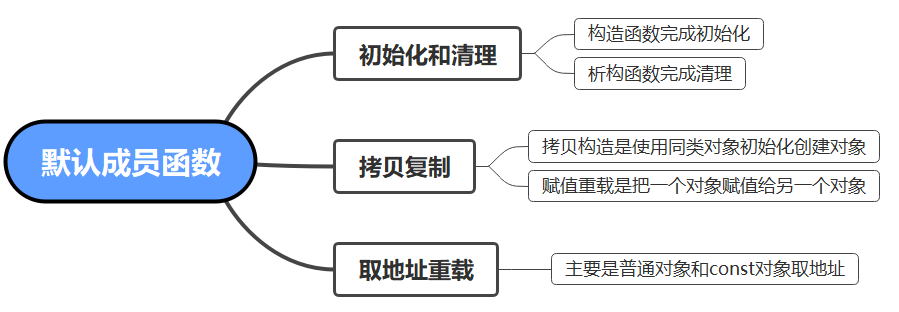## 构造函数

### 构造函数概念

☀️和上次一样，首先呢，我们先创建一个Date类。

``class Date{public:    void SetDate(int year, int month, int day){ _year = year; _month = month; _day = day;    } void Print(){ cout << _year << "-" << _month << "-" << _day << endl;    }private:int _year;int _month;int _day;    }````class Date{public:    Date(int year, int month, int day){ _year = year; _month = month; _day = day;    } void Print(){ cout << _year << "-" << _month << "-" << _day << endl;    }private:    int _year;    int _month;    int _day;};int main(){    Date a(2022, 5, 20);    a.Print();  // ---> 2022-5-20    return 0;}``

### 构造函数特点

1. 函数名与类名相同

2. 无返回值

3. 对象创建时编译器自动调用相对应的构造函数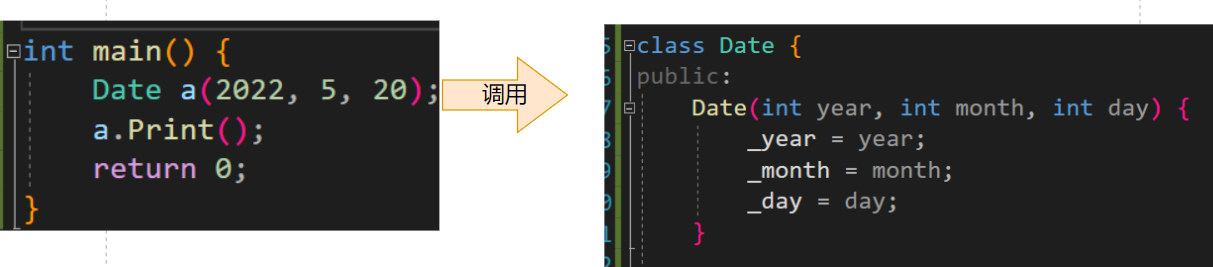4. 构造函数可以重载

我们也可以在类中写几个不同的构造函数，来面对不同的参数传递方式。

``class Date{public:    Date(int year, int month, int day){ _year = year; _month = month; _day = day;    } Date(){ _year = 2021; _month = 1; _day = 1;    } void Print(){ cout << _year << "-" << _month << "-" << _day << endl;    }private:    int _year;    int _month;    int _day;};int main(){    Date a(2022, 5, 20);    Date b;    a.Print(); // --->2022-5-20    b.Print(); // --->2021-1-1    return 0;}``

调用方式如下：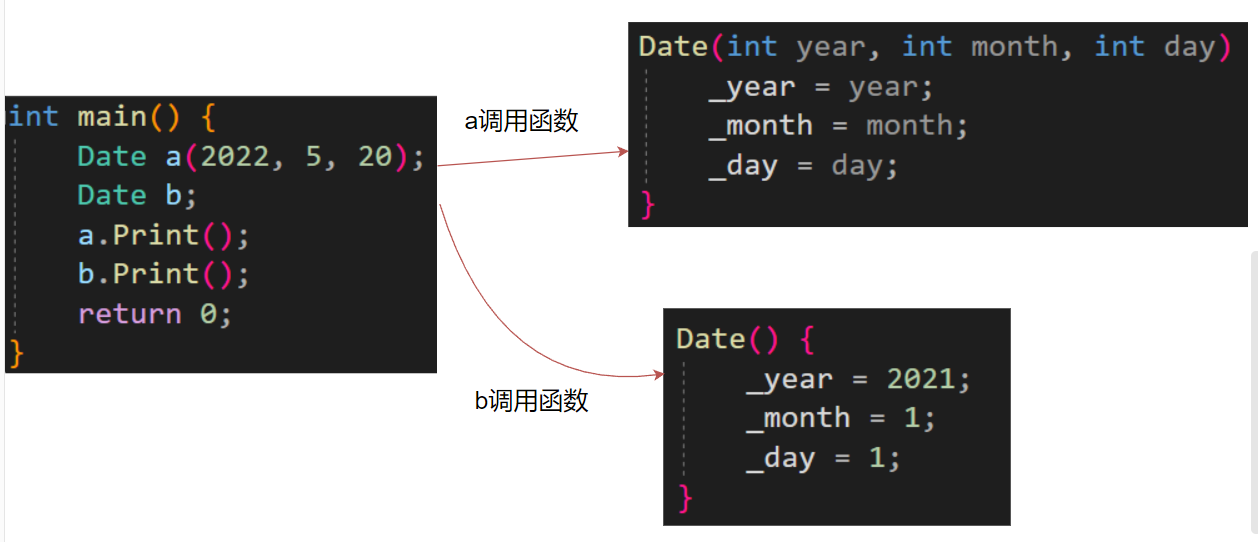5. 当我们未定义显示的构造函数时，编译器会自动生成一个无参的默认构造函数，一旦用户显示定义编译器将不再生成

如果我们将原有的构造函数删掉，

``class Date{public: void Print(){ cout << _year << "-" << _month << "-" << _day << endl;    }private:    int _year;    int _month;    int _day;};int main(){    Date b;    b.Print();  //-858993460--858993460--858993460    return 0;}``

我们发现b的内容为随机值，那么这是什么原因呢？

默认的构造函数对我们不同的成员变量采取不同的处理方式。

• 内置类型如（int，char，double。。。等C++中本身存在的类型）不进行初始化
• 自定义类型（class，struct等）会去调用此类型的构造函数

我们用下面的例子来解释一下。

``//栈类class Stack {public:Stack() {arr = (int*)malloc(sizeof(int) * 10);top = 0;}private:int top;int* arr;};//两个栈类和一个队头来组成队列类class Queue {int head;Stack _a1;Stack _a2;};``

创建方式如下图：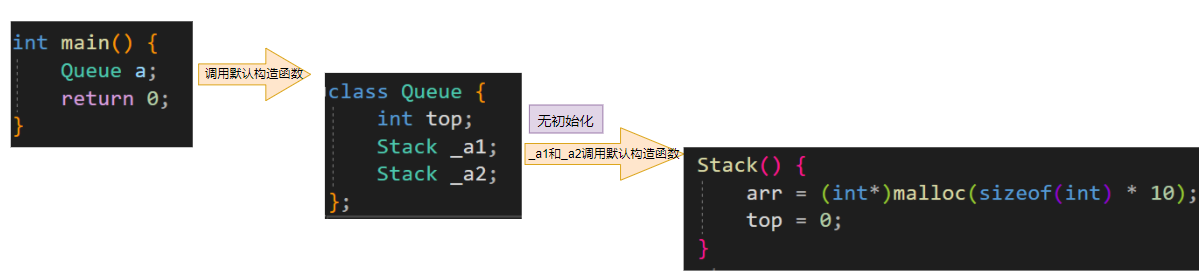6. 无参的构造函数和全缺省的构造函数都称为默认构造函数，并且默认构造函数只能有一个。注意：无参构造函数、全缺省构造函数、我们没写编译器默认生成的构造函数，都可以认为是默认成员函数。

简单来说，就是我们在调用的时候无需传递参数的构造函数，就是默认成员函数。

## 析构函数

### 析构函数的概念

``class Stack {public:Stack() {arr = (int*)malloc(sizeof(int) * 10);top = 0;}private:int top;int* arr;};``

``class Stack {public:Stack() {arr = (int*)malloc(sizeof(int) * 10);top = 0;}    //析构函数与构造函数大体相似，在函数名前加上~即可    ~Stack(){ free(arr);    }private:int top;int* arr;};``

### 析构函数特性

1. 析构函数名是在类名前加上字符’~'。

2. 无参数无返回值

3. 一个类只有一个析构函数。如未显示定义，系统会自动生成默认析构函数。

默认的析构函数和我们之前学习的编译器自己创建的默认构造函数作用相似。

• 内置类型直接销毁。
• 自定义类型会去它自己的析构函数。
4. 对象生命周期结束时，C++编译器自动调用析构函数。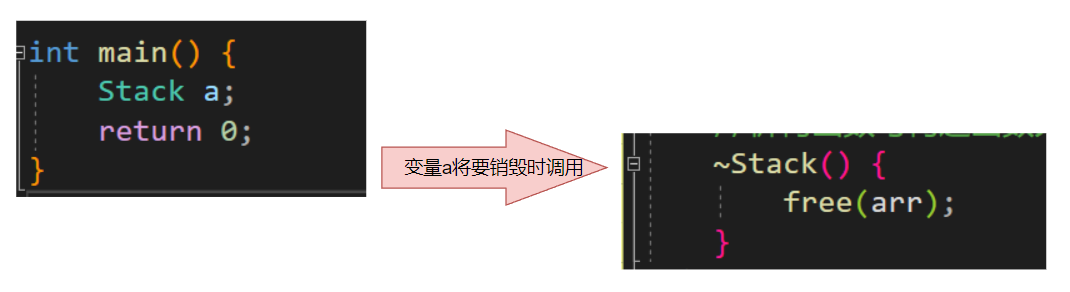## 拷贝构造

``class Date{public:    Date(int year, int month, int day){ _year = year; _month = month; _day = day;    } Date(const Date& d){  //拷贝构造函数 _year = d._year; _month = d._month; _day = d._day;    } void Print(){ cout << _year << "-" << _month << "-" << _day << endl;    }private:    int _year;    int _month;    int _day;};int main(){    Date a(2022, 5, 20);    Date b(a);      //此时就可以调用我们的拷贝构造了    b.Print();      //---->2022-5-20    return 0;}``

### 拷贝构造函数特点

1. 拷贝构造函数是构造函数的一个重载形式

拷贝构造函数本质上就是我们的构造函数，只是传递的参数发生了改变。

2. 拷贝构造函数的参数只有一个必须使用引用传参，使用传值方式会引发无穷递归调用

首先我们要知道一点，C++中的传参遵循以下规则：

1. 如果是内置类型传参时是直接将实参的值按字节拷贝到参数中。
2. 自定义类型在传参时会去调用此类型的拷贝构造函数。

接下来我们来解释一下为什么会发生无穷递归调用。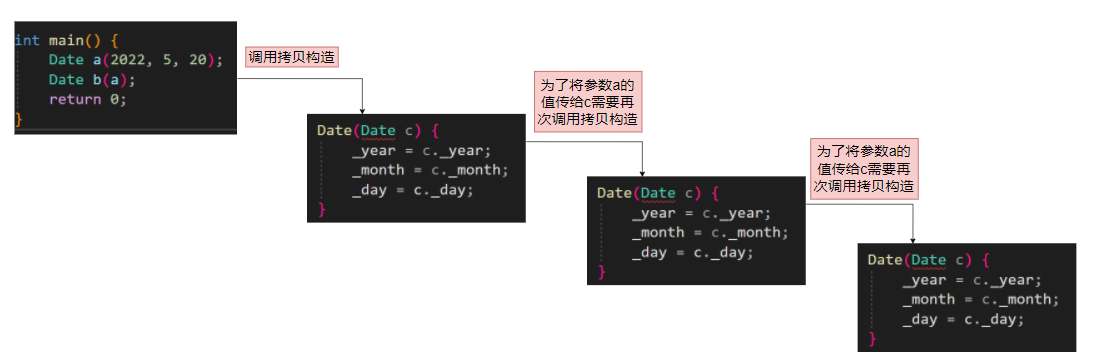就像上图一样为了将我们的参数传给函数会不断的去调用拷贝构造函数，这就会导致死递归。

3. 若未显式定义，系统生成默认的拷贝构造函数。 默认的拷贝构造函数对象按内存存储按字节序完成拷贝，这种拷贝我们叫做浅拷贝，或者值拷贝。

比如我们写的Date类，如果我们不写，使用编译器默认生成的拷贝构造函数依然可以实现拷贝构造。但是如果类中需要资源管理，那么默认生成的拷贝构造函数就有可能产生问题。

还是使用我们之前的Queue类来解释这个问题。

``//栈类class Stack {public:    //构造函数Stack() {arr = (int*)malloc(sizeof(int) * 10);top = 0;}    //析构函数     ~Stack(){ free(arr);    }private:int top;int* arr;};int main(){    Stack a;    Stack b(a);     //调用拷贝构造    return 0;}``

在上面的代码中，我们首先创建了一个对象a，然后又有编译器默认生成的拷贝构造函数去生成了b。此时a和b中的top变量相同并且arr指针指向同一块空间。目前为止好像并没有什么问题。但当我们运行起来时发现：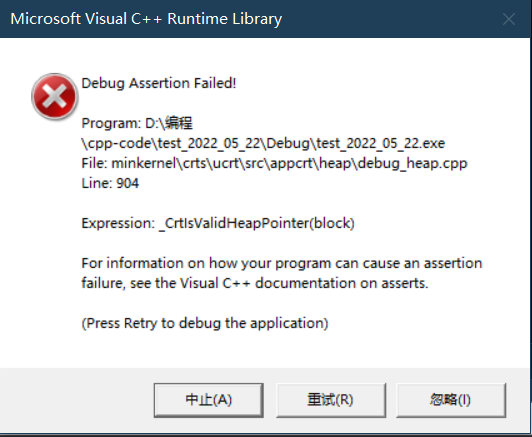**程序崩溃了！！！**这是为什么呢？因为a和b中的arr指针都指向同一片空间，当程序结束去调用析构函数时，开辟的那一片空间会被free两次，这就是问题的所在，而为了解决这个问题呢，就需要用到深拷贝了。深拷贝我们以后再聊。

## 运算符重载

C++为了增强代码的可读性引入了运算符重载，运算符重载是具有特殊函数名的函数，也具有其返回值类型，函数名字以及参数列表，其返回值类型与参数列表与普通的函数类似。

``书写方式：返回类型 operator 需要重载的运算符 （参数列表）``

1. 不能通过连接其他符号来创建新的操作符：比如operator@ 。
2. 重载操作符必须有一个类类型或者枚举类型的操作数。
3. 用于内置类型的操作符，其含义不能改变，例如：内置的整型+，不 能改变其含义。
4. 作为类成员的重载函数时，其形参看起来比操作数数目少1成员函数的操作符有一个默认的形参this，限定为第一个形参。
5. .* 、:: 、sizeof 、?: 、. 注意以上5个运算符不能重载。

### 重载运算符的使用

``class Date{public:    Date(int year, int month, int day){ _year = year; _month = month; _day = day;    } void Print(){ cout << _year << "-" << _month << "-" << _day << endl;    }private:    int _year;    int _month;    int _day;};int main(){    Date d1(2022,5,21);    Date d2(2022,5,22);    return 0;}``

``class Date{public:    Date(int year, int month, int day){ _year = year; _month = month; _day = day;    }    bool operator >(Date& d){ if(_year > d._year ||    _year == d._year && _month > d._month ||    _year == d._year && _month == d._month && _day > d._day){     return true; } else{     return false; }    } void Print(){ cout << _year << "-" << _month << "-" << _day << endl;    }private:    int _year;    int _month;    int _day;};``

``int main(){    Date a(2022,5,20);    Date b(2022,5,21);    if (b > a){ cout << "大于" << endl;    }    else{ cout << "小于等于" << endl;    }return 0;}``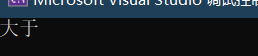## 拷贝构造和“=”重载区别

``//首先还是一个Date类class Date{public:    Date(int year = 1, int month = 1, int day = 1){ _year = year; _month = month; _day = day;    } Date(const Date& b){ cout << "拷贝构造" << endl; _year = b._year; _month = b._month; _day = b._day;    } Date& operator=(const Date& b){ cout << "运算符重载" << endl; _year = b._year; _month = b._month; _day = b._day; return *this;}private:int _year;    int _month;    int _day;};int main(){    Date a(2022, 5, 20);    Date b = a;    //(1)    Date c;    c = a;  //(2)}``

（1）处调用的是拷贝构造还是运算符重载呢？

（2）处调用的是拷贝构造还是运算符重载呢？b和c二者的区别在于，一个是在创建变量时进行初始化，一个是变量创建完毕后进行赋值。所以在定义对象进行赋值时会调用拷贝构造，而已经定义好的变量去赋值的时候会调用运算符重载。

## 结语